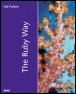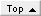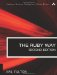# Working with GraphsRuby Way By Hal Fulton Slots : 1.0 Table of Contents

A graph is a collection of nodes that interconnect with each other arbitrarily. (A tree is a special case of a graph.) We will not get deeply into graphs because the theory and terminology can have a steep learning curve. Before long, we would find ourselves wandering out of the field of computer science entirely and into the province of mathematicians.

Yet, graphs do have many practical applications. Consider any ordinary highway map, with highways connecting cities, or consider a circuit diagram. These are both best represented as graphs. A computer network can be thought of in terms of graph theory, whether it is a LAN of a dozen systems or the Internet itself with its countless millions of nodes.

When we say "graph," we usually mean an undirected graph. In simplistic terms, this is a graph in which the connecting lines don't have arrows; two nodes are either connected or they are not. By contrast, a directed graph or digraph can have "one-way streets;" just because node x is connected to node y doesn't mean that the reverse is true. (A node is also commonly called a vertex.) Finally, a weighted graph has connections (or edges) that have weights associated with them; these weights may express, for instance, the "distance" between two nodes. We won't go beyond these basic kinds of graphs; if you're interested in learning more, you can refer to the numerous references in computer science and mathematics.

In Ruby, as in most languages, a graph can be represented in multiple waysfor example, as a true network of interconnected objects or as a matrix storing the set of edges in the graph. We will look at both of these as we show a few practical examples of manipulating graphs.

#### Implementing a Graph As an Adjacency Matrix

The example here builds on two previous examples. In Listing 3.21, we implement an undirected graph as an adjacency matrix, using the ZArray class to make sure new elements are zero and inheriting from TriMatrix to get a lower triangular matrix form.

Note that in the kind of graph we are implementing here, a node cannot be connected to itself, and two nodes can be connected by only one edge.

We provide a way to specify edges initially by passing pairs into the constructor. We also provide a way to add and remove edges and detect the presence of edges. The vmax method will return the highest-numbered vertex in the graph. The degree method will find the degree of the specified vertex (that is, the number of edges that connect to it).

Finally, we provide two iterators, each_vertex and each_edge. These will iterate over vertices and edges, respectively.

` class LowerMatrix < TriMatrix   def initialize     @store = ZArray.new   end end class Graph   def initialize(*edges)     @store = LowerMatrix.new     @max = 0     for e in edges       e, e = e, e if e > e       @store[e,e] = 1       @max = [@max, e, e].max     end   end def [](x,y)   if x > y     @store[x,y]   elsif x < y     @store[y,x]   else     0   end end def []=(x,y,v)   if x > y     @store[x,y]=v   elsif x < y     @store[y,x]=v   else     0   end end def edge? x,y   x,y = y,x if x < y   @store[x,y]==1 end def add x,y   @store[x,y] = 1 end def remove x,y   x,y = y,x if x < y   @store[x,y] = 0   if (degree @max) == 0     @max -= 1   end end   def vmax     @max   end   def degree x     sum = 0     0.upto @max do |i|       sum += self[x,i]     end     sum   end   def each_vertex     (0..@max).each { |v| yield v}   end   def each_edge     for v0 in 0..@max       for v1 in 0..v0-1         yield v0,v1 if self[v0,v1]==1       end     end   end end mygraph = Graph.new([1,0],[0,3],[2,1],[3,1],[3,2]) # Print the degrees of all the vertices: 2 3 3 2 mygraph.each_vertex { |v| puts mygraph.degree(v)} # Print the list of edges mygraph.each_edge do |a,b|   puts "(#{ a} ,#{ b} )" end # Remove a single edge mygraph.remove 1,3 # Print the degrees of all the vertices: 2 2 2 2 mygraph.each_vertex { |v| p mygraph.degree v} `

#### Determining Whether a Graph Is Fully Connected

Not all graphs are fully connected. That is, sometimes "you can't get there from here" (there may be vertices that are unreachable from other vertices no matter what path you try). Connectivity is an important property of a graph to be able to assess, telling whether the graph is "of one piece." If it is, every node is ultimately reachable from every other node.

We won't explain the algorithm; you can refer to any discrete math book. However, we offer the Ruby method in Listing 3.22.

##### Listing 3.22 Determining Whether a Graph Is Fully Connected
` class Graph   def connected?     x = vmax     k = [x]     l = [x]     for i in 0..@max       l << i if self[x,i]==1     end     while !k.empty?       y = k.shift       # Now find all edges (y,z)       self.each_edge do |a,b|         if a==y || b==y           z = a==y ? b : a           if !l.include? z             l << z             k << z           end         end       end     end     if l.size < @max       false     else       true     end   end end mygraph = Graph.new([0,1], [1,2], [2,3], [3,0], [1,3]) puts mygraph.connected?     # true puts mygraph.euler_path?    # true mygraph.remove 1,2 mygraph.remove 0,3 mygraph.remove 1,3 puts mygraph.connected?     # false puts mygraph.euler_path?    # false `

A refinement of this algorithm could be used to determine the set of all connected components (or cliques) in a graph that is not overall fully connected. We won't do this here.

#### Determining Whether a Graph Has an Euler Circuit

There is no branch of mathematics, however abstract, which may not some day be applied to phenomena of the real world.

Nikolai Lobachevsky

Sometimes we want to know whether a graph has an Euler circuit. This term comes from the mathematician Leonhard Euler who essentially founded the field of topology by dealing with a particular instance of this question. (A graph of this nature is sometimes called a unicursive graph because it can be drawn without lifting the pen from the paper or retracing.)

In the German town of Königsberg is an island in the middle of a river (near where the river splits into two parts). Seven bridges crisscross at various places between opposite shores and the island. The townspeople wondered whether it was possible to make a walking tour of the city in such a way that you would cross each bridge exactly once and return to your starting place. In 1735, Euler proved that this was impossible. This, then, is not just a classic problem, but the original graph theory problem.

And, as with many things in life, once you know the answer, it is easy. It turns out that for a graph to have an Euler circuit, it must possess only vertices with even degree. Here, we add a little method to check that property:

` `

` class Graph   def euler_circuit?     return false if !connected?     for i in 0..@max       return false if degree(i) % 2 != 0     end     true   end end mygraph = Graph.new([1,0],[0,3],[2,1],[3,1],[3,2]) flag1 =  mygraph.euler_circuit?    # false mygraph.remove 1,3 flag2 =  mygraph.euler_circuit?    # true `

#### Determining Whether a Graph Has an Euler Path

An Euler path is not quite the same as an Euler circuit. The word circuit implies that you must return to your starting point; with a path, we are really only concerned with visiting each edge exactly once. The following code fragment illustrates the difference:

` `

` class Graph   def euler_path?     return false if !connected?     odd=0     each_vertex do |x|       if degree(x) % 2 == 1         odd += 1       end     end     odd <= 2   end end mygraph = Graph.new([0,1],[1,2],[1,3],[2,3],[3,0]) flag1 =  mygraph.euler_circuit?    # false flag2 =  mygraph.euler_path?       # true `

#### Hints for More Complex Graphs

It would be possible to write an entire book about graph algorithms. There are many good ones out there already, and we are certainly not going to range that far outside our realm of expertise.

However, we will offer a few hints for dealing with more sophisticated graphs. These hints should get you started if you need to tackle a more advanced problem.

Suppose you want a directed graph rather than an undirected one. Just because vertex x points to vertex y doesn't mean the converse is true. You should no longer use a lower triangular matrix form but rather a full-fledged two-dimensional array (see the section "Using Multidimensional Arrays"). You may still find ZArray useful (see the section "Establishing a Default Value for New Array Elements"). You will likely want to implement not just a degree method but rather a pair of them; in a directed graph, a vertex has an in-degree and an out-degree.

Suppose you have a directed graph in which a node or vertex is allowed to point to itself. Now you have potential nonzero numbers in the diagonal of your matrix rather than just zeroes. Be sure your code doesn't disallow access to the diagonal.

Suppose you want a weighted graph, where each edge has a weight associated with it. Now you would store the weight itself in the array rather than just a 1 or 0 (present or absent).

What about a multigraph, in which there can be multiple connections (edges) between the same pair of vertices? If it is undirected, a lower triangular matrix will suffice, and you can store the number of edges in each element (rather than just a 1 or 0). If it is directed, you will need a two-dimensional array, and you can still store the number of edges in each respective element.

What about bizarre combinations of these? For example, it is certainly conceivable to have a weighted, directed multigraph (and if you have a valid everyday need for one, let us know about your application). In this case, you would need a more complex data structure. One possibility would be to store a small array in each element of the matrix.

For example, suppose vertex 3 has five edges connecting it with vertex 4; then element 3,4 of the adjacency matrix might store an array containing the associated weights.

The possibilities are endless and are beyond the scope of this book.The Ruby Way, Second Edition: Solutions and Techniques in Ruby Programming (2nd Edition)
ISBN: 0672328844
EAN: 2147483647
Year: 2000
Pages: 119
Authors: Hal Fulton

Similar book on Amazon